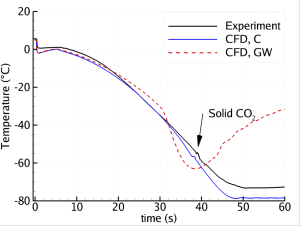# Figure 2: Depressurization of a 200 m pipe: Temperature plotted versus time at a position 195 m from the outlet (which is 5 m from the closed end). Experimental data compared to our CFD calculations using two different heat-transfer models.Figure 2: Depressurization of a 200 m pipe: Temperature plotted versus time at a position 195 m from the outlet (which is 5 m from the closed end). Experimental data compared to our CFD calculations using two different heat-transfer models.Figure 2: Depressurization of a 200 m pipe: Temperature plotted versus time at a position 195 m from the outlet (which is 5 m from the closed end). Experimental data compared to our CFD calculations using two different heat-transfer models.